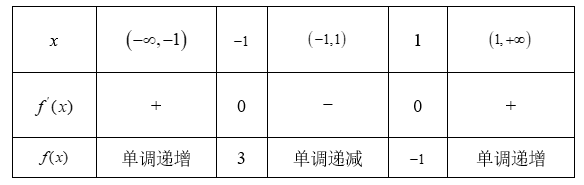(1) 求函数 $y=f(x)$ 在点 $(0, f(0))$ 处的切线方程;
(2) 求函数 $f(x)$ 的极值.

\begin{aligned} &\therefore f^{\prime}(x)=3 x^{2}-3=3(x-1)(x+1), \\ &\therefore k=f^{\prime}(0)=-3 \text {, 又 } f(0)=1 \end{aligned}

(2) $f^{\prime}(x)=3 x^{2}-3=3(x-1)(x+1)$①点击 首页查看更多试卷和试题 , 点击查看 本题所在试卷